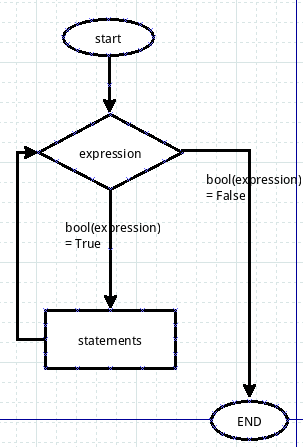AI教程网 - 未来以来，拥抱AI；新手入门，从AI教程网开始......

# 零基础学Python:语句(5)

131℃

while，翻译成中文是“当…的时候”，这个单词在英语中，常常用来做为时间状语，while … someone do somthing，这种类型的说法是有的。在python中，它也有这个含义，不过有点区别的是，“当…时候”这个条件成立在一段范围或者时间间隔内，从而在这段时间间隔内让python做好多事情。就好比这样一段情景：

``````while 年龄大于60岁：-------->当年龄大于60岁的时候
退休            -------->凡是符合上述条件就执行的动作
``````## 做猜数字游戏

``````#! /usr/bin/env python
#coding:UTF-8

import random

i=0
while i < 4:
print'********************************'
num = input('请您输入0到9任一个数：')       #李同学用的是python3

xnum = random.randint(0,9)

x = 3 - i

if num == xnum:
print'运气真好，您猜对了！'
break
elif num > xnum:
print'''您猜大了!\n哈哈,正确答案是:%s\n您还有%s次机会！''' %(xnum,x)
elif num < xnum:
print'''您猜小了!\n哈哈,正确答案是:%s\n您还有%s次机会！''' %(xnum,x)
print'********************************'

i += 1
``````

``````num_input = raw_input("please input one integer that is in 1 to 100:")    #我用的是python2.7，在输入指令上区别于李同学
``````

1. 输入的是否是整数
2. 如果是整数，是否在1到100之间。

``````if not num_input.isdigit():     #str.isdigit()是用来判断字符串是否纯粹由数字组成
elif int(num_input)<0 and int(num_input)>=100:
print "The number should be in 1 to 100."
else:
pass       #这里用pass，意思是暂时省略，如果满足了前面提出的要求，就该执行此处语句
``````

``````import random

number = random.randint(1,100)

while True:             #不限制用户的次数了
...
``````

``````#!/usr/bin/env python
#coding:utf-8

import random

number = random.randint(1,101)

guess = 0

while True:

num_input = raw_input("please input one integer that is in 1 to 100:")
guess += 1

if not num_input.isdigit():
elif int(num_input) < 0 or int(num_input) >= 100:
print "The number should be in 1 to 100."
else:
if number == int(num_input):
print "OK, you are good.It is only %d, then you successed." % guess
break
elif number > int(num_input):
print "your number is more less."
elif number < int(num_input):
else:
print "There is something bad, I will not work"
``````

## break和continue

break,在上面的例子中已经出现了，其含义就是要在这个地方中断循环，跳出循环体。下面这个简要的例子更明显：

``````#!/usr/bin/env python
#coding:utf-8

a = 8
while a:
if a%2 == 0:
break
else:
print "%d is odd number"%a
a = 0
print "%d is even number"%a
``````

a=8的时候，执行循环体中的break，跳出循环，执行最后的打印语句，得到结果：

``````8 is even number
``````

``````9 is odd number
0 is even number
``````

``````#!/usr/bin/env python
#coding:utf-8

a = 9
while a:
if a%2==0:
a -=1
continue    #如果是偶数，就返回循环的开始
else:
print "%d is odd number"%a #如果是奇数，就打印出来
a -=1
``````

## while…else

``````#!/usr/bin/env python

count = 0
while count < 5:
print count, " is  less than 5"
count = count + 1
else:
print count, " is not less than 5"
``````

``````0 is less than 5
1 is less than 5
2 is less than 5
3 is less than 5
4 is less than 5
5 is not less than 5
``````

## for…else

``````#!/usr/bin/env python
# coding=utf-8

from math import sqrt

for n in range(99, 1, -1):
root = sqrt(n)
if root == int(root):
print n
break

else:
print "Nothing."
``````